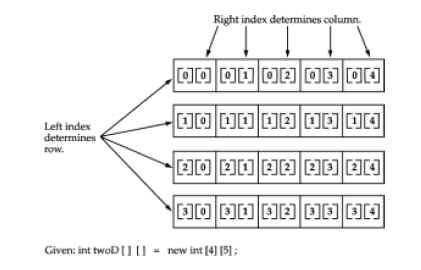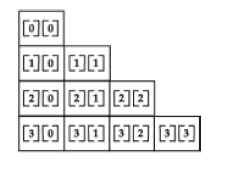# Programming Tutorials

## Multidimensional or arrays of arrays in Java

By: Abinaya in Java Tutorials on 2007-09-07

In Java, multidimensional arrays are actually arrays of arrays. These, as you might expect, look and act like regular multidimensional arrays. However, as you will see, there are a couple of subtle differences. To declare a multidimensional array variable, specify each additional index using another set of square brackets. For example, the following declares a two-dimensional array variable called twoD. `int twoD[][] = new int;`

This allocates a 4 by 5 array and assigns it to twoD. Internally this matrix is implemented as an array of arraysof int. Conceptually, this array will look like the one shown in Figure below:The following program numbers each element in the array from left to right, top to bottom, and then displays these values:

```// Demonstrate a two-dimensional array.
class TwoDArray {
public static void main(String args[]) {
int twoD[][] = new int;
int i, j, k = 0;
for (i = 0; i < 4; i++)
for (j = 0; j < 5; j++) {
twoD[i][j] = k;
k++;
}
for (i = 0; i < 4; i++) {
for (j = 0; j < 5; j++)
System.out.print(twoD[i][j] + " ");
System.out.println();
}
}
}```

This program generates the following output:

`0 1 2 3 45 6 7 8 910 11 12 13 1415 16 17 18 19`

When you allocate memory for a multidimensional array, you need only specify the memory for the first (leftmost) dimension. You can allocate the remaining dimensions separately. For example, this following code allocates memory for the first dimension of twoD when it is declared. It allocates the second dimension manually.

`int twoD[][] = new int[];twoD = new int;twoD = new int;twoD = new int;twoD = new int;`

While there is no advantage to individually allocating the second dimension arrays in this situation, there may be in others. For example, when you allocate dimensions manually, you do not need to allocate the same number of elements for each dimension. As stated earlier, since multidimensional arrays are actually arrays of arrays, the length of each array is under your control. For example, the following program creates a two-dimensional array in which the sizes of the second dimension are unequal.

```// Manually allocate differing size second dimensions.
class TwoDAgain {
public static void main(String args[]) {
int twoD[][] = new int[];
twoD = new int;
twoD = new int;
twoD = new int;
twoD = new int;
int i, j, k = 0;
for (i = 0; i < 4; i++)
for (j = 0; j < i + 1; j++) {
twoD[i][j] = k;
k++;
}
for (i = 0; i < 4; i++) {
for (j = 0; j < i + 1; j++)
System.out.print(twoD[i][j] + " ");
System.out.println();
}
}
}```

This program generates the following output:

`01 23 4 56 7 8 9`

The array created by this program looks like this:The use of uneven (or, irregular) multidimensional arrays is not recommended for most applications, because it runs contrary to what people expect to find when a multidimensional array is encountered. However, it can be used effectively in some situations. For example, if you need a very large two-dimensional array that is sparsely populated (that is, one in which not all of the elements will be used), then an irregular array might be a perfect solution.

It is possible to initialize multidimensional arrays. To do so, simply enclose each dimension's initializer within its own set of curly braces. The following program creates a matrix where each element contains the product of the row and column indexes. Also notice that you can use expressions as well as literal values inside of array initializers.

```// Initialize a two-dimensional array.
class Matrix {
public static void main(String args[]) {
double m[][] = {
{ 0 * 0, 1 * 0, 2 * 0, 3 * 0 },
{ 0 * 1, 1 * 1, 2 * 1, 3 * 1 },
{ 0 * 2, 1 * 2, 2 * 2, 3 * 2 },
{ 0 * 3, 1 * 3, 2 * 3, 3 * 3 }
};
int i, j;
for (i = 0; i < 4; i++) {
for (j = 0; j < 4; j++)
System.out.print(m[i][j] + " ");
System.out.println();
}
}
}```

When you run this program, you will get the following output:

`0.0 0.0 0.0 0.00.0 1.0 2.0 3.00.0 2.0 4.0 6.00.0 3.0 6.0 9.0`

As you can see, each row in the array is initialized as specified in the initialization lists. Let's look at one more example that uses a multidimensional array. The following program creates a 3 by 4 by 5, three-dimensional array. It then loads each element with the product of its indexes. Finally, it displays these products.

```// Demonstrate a three-dimensional array.
class threeDMatrix {
public static void main(String args[]) {
int threeD[][][] = new int;
int i, j, k;
for (i = 0; i < 3; i++)
for (j = 0; j < 4; j++)
for (k = 0; k < 5; k++)
threeD[i][j][k] = i * j * k;
for (i = 0; i < 3; i++) {
for (j = 0; j < 4; j++) {
for (k = 0; k < 5; k++)
System.out.print(threeD[i][j][k] + " ");
System.out.println();
}
System.out.println();
}
}
}```

This program generates the following output:

`0 0 0 0 00 0 0 0 00 0 0 0 00 0 0 0 00 0 0 0 00 1 2 3 40 2 4 6 80 3 6 9 120 0 0 0 00 2 4 6 80 4 8 12 160 6 12 18 24`